# ===Open Problem #4===

Welcome to Open Problem #4! This is the first problem that's been drawn from our suggestion thread. This problem comes from Andrew Hayes. I want to draw from that thread more, so please, if you have any ideas for future open problems, send them in!

Consider an $n$ by $n$ grid. Each square can either be open or closed, except for the top left and bottom right squares, which are always open. How many arrangements exist such that there exists a path from the top left to the bottom right square (moving only up/down/left/right)?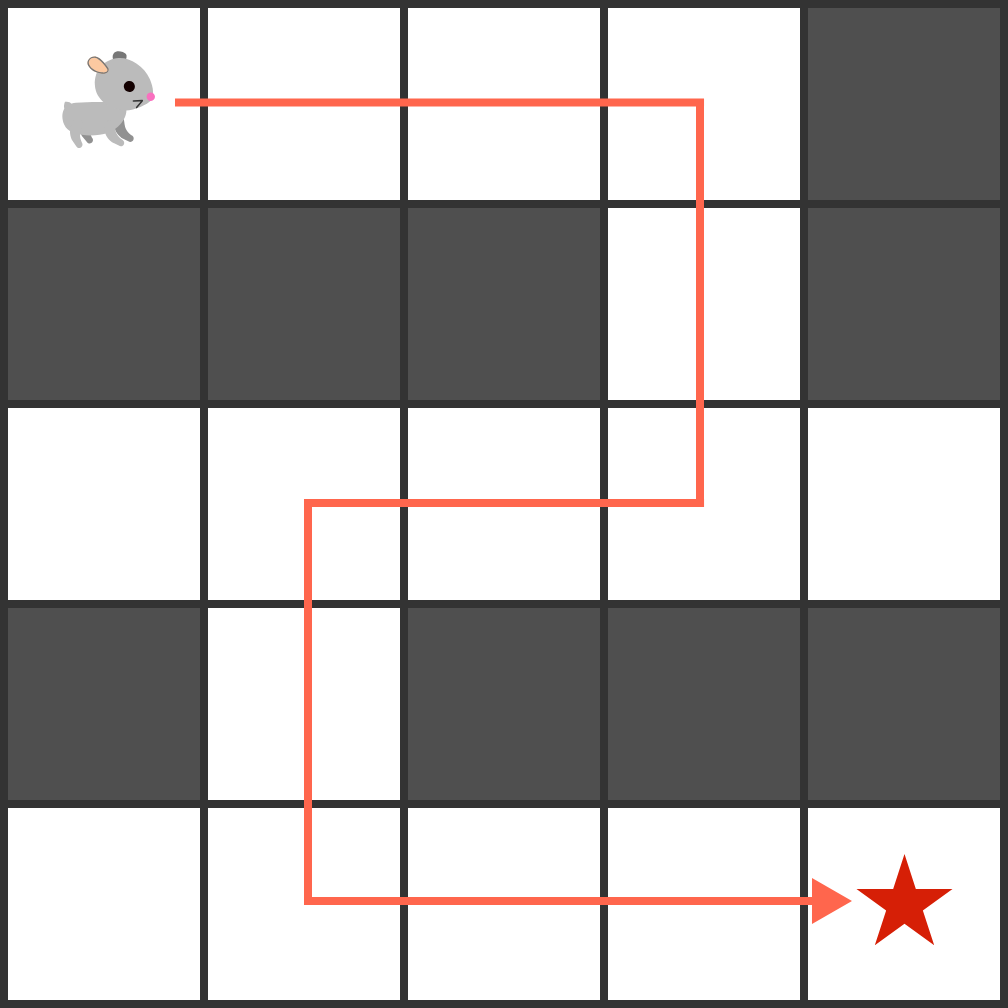Computer science mavens may recognize this as related to the "Rat in a Maze" problem used in textbooks. In the traditional setup, you are given the maze and then asked how many solutions are there. The textbook question is then to write a backtracking algorithm that finds all solutions to the maze.

The question posed here is very different, and as far as I can find in my comprehensive search, has never been asked. If we are given a particular size of grid, how many unique "mazes" can we make? Note we could adapt existing source code (like the example above) to work on solving this by simply adding a loop that tests every single maze configuration for a particular size. This is actually a fairly good way to start the problem.

However, we'd like to go a little farther, and start finding mathematical patterns. One approach might be to only ask about how many "mazes of a particular type" there might be; so not a comprehensive counting, but using some sort of generating rule to make mazes that are guaranteed to work and where it's easy to count how many mazes come from the rule.

As a goal for this open question, I'd like to get enough information that we can make the start of an entry in the On-Line Encyclopedia of Integer Sequences. (That is, we can solve it for 2 by 2, 3 by 3, 4 by 4 etc. up to a certain point - even if we don't have a general formula, we can calculate as many numbers as possible in the sequence.)

Other news:

For Open Problem #3, if you haven't read it yet I definitely recommend David Vreken's solution here. He has two more example figures and mentions a possible new direction for research (using shapes other than isosceles right triangles for the "folding" method).

I will be putting the paper from Open Problem #2 up on ArXiv fairly soon, and sending it somewhere for publication (I'm guessing by the end of this week).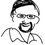Note by Jason Dyer
3 years, 2 months ago

This discussion board is a place to discuss our Daily Challenges and the math and science related to those challenges. Explanations are more than just a solution — they should explain the steps and thinking strategies that you used to obtain the solution. Comments should further the discussion of math and science.

When posting on Brilliant:

• Use the emojis to react to an explanation, whether you're congratulating a job well done , or just really confused .
• Ask specific questions about the challenge or the steps in somebody's explanation. Well-posed questions can add a lot to the discussion, but posting "I don't understand!" doesn't help anyone.
• Try to contribute something new to the discussion, whether it is an extension, generalization or other idea related to the challenge.

MarkdownAppears as
*italics* or _italics_ italics
**bold** or __bold__ bold
- bulleted- list
• bulleted
• list
1. numbered2. list
1. numbered
2. list
Note: you must add a full line of space before and after lists for them to show up correctly
paragraph 1paragraph 2

paragraph 1

paragraph 2

[example link](https://brilliant.org)example link
> This is a quote
This is a quote
    # I indented these lines
# 4 spaces, and now they show
# up as a code block.

print "hello world"
# I indented these lines
# 4 spaces, and now they show
# up as a code block.

print "hello world"
MathAppears as
Remember to wrap math in $$ ... $$ or $ ... $ to ensure proper formatting.
2 \times 3 $2 \times 3$
2^{34} $2^{34}$
a_{i-1} $a_{i-1}$
\frac{2}{3} $\frac{2}{3}$
\sqrt{2} $\sqrt{2}$
\sum_{i=1}^3 $\sum_{i=1}^3$
\sin \theta $\sin \theta$
\boxed{123} $\boxed{123}$

Sort by:

A few more thoughts, without trying to give exact results: 1. Let the board be n cells by n cells and consider a large sample of configurations in which each cell has half a chance of being open = white or closed = black, except for the north-west cell. In the original problem the south-east square is also open, and in this case define P[n] to be the probablity of there being a path between these two squares moving only horizontally or vertically between adjacent open squares. If the south-east square only has half a chance of being open, define R[n] to be the probability of a path between the two squares and note that P[n] = 2 R[n]. 2. Now let the board be semi-infinite: that is let the board have north-west square with coordinates (1,1) [not (0,0) as in my earlier posts] and extend infinitely far to the south and east. Again consider a large sample of configurations in which each cell has half a chance of being open or closed apart from the north-west square which is always open. Let Q[n] be the probability of a path from this square to the square (n,n). By experiment, we find that Q[n] is roughly about twice R[n]. In other words, there are many paths which go beyond the cells with x or y coordinates equal to n, but double back to reach the square (n,n). {It remains true that Q[n] and R[n] are small in absolute size.} 3. On this semi-infinite board, define D[n] to be the probability of a path from the north-west square reaching any cell (x,y) on the cross diagonal, where x+y = n + 1. 4. By experiment, on values of n up to about 50, we find R[n+1] / R[n] -> a constant around 0.7 to 0.8, with similar results for P, Q and D, where the constant has a similar but different value. Comments:- A) (4) implies that the number of paths tends to K.( 2^(n^2 - 2 ) ).(k^n), where K and k are constants. B) (2) suggests that a more natural problem is to find the probability on semi-infinite board or one which is infinite in all directions. C) I have tried normalising the probabilities R[n] by the total on the the diagonal, D[n], and comparing it with the values in Pascal's triangle, without getting any decisive result. However, I think there must be something in this approach, especially as Pascal's triangle is related to the number of paths from the north-west square to the another given square. It would be natural to extend the notion of Pascal's triangle by including paths which turn back on themselves.

- 3 years, 1 month ago

Some more statistics from an experiment with a board size 50 x 50:- A sample of 1000 million [ = one billion ] configurations was generated at random. In each trial, each square had exactly half a chance of being open or closed, [as computed the java Random class] except for the top left and bottom right squares that were always open. In this sample, 534 configurations had a path from top left to bottom right, [moving up, down or side ways, but not diagonally] along open squares. Thus configurations with paths are very rare. Amongst all configurations in the sample, the mean number of open squares in the island of open squares including the top left square and connected by moves as described was 11.781. Let the top left hand square have (x,y) coordinates (0, 0), so that its neighbor to the right has coordinates (1,0) and its neighbor below has coordinates (0,1). For each of these islands define "the furthest square reached" to be the square in the island for which the sum, S, of its x and y coordinates is the greatest. Then for each island, define S to be "the furthest distance reached". In the sample the mean of "the furthest distance reached" over all islands was 4.6768. Again I find this number surprisingly small.

- 3 years, 2 months ago

If you take a large number of random configurations of open/closed squares for any board of moderate size (anything over n = 20) will do, then the mean size of the island consisting of connected open squares which includes the top left hand square is between 11 and 12. By the time n = 50, mean size of this island has settled down to its asymptotic value of 11.78.

- 3 years, 2 months ago

Since my comment yesterday, I have been doing a bit of experimenting with random configurations, of size n x n ~ 30x30. It is clear that as n tends to infinity the proportion of configurations with a path top left to bottom right (TL - BR) tends to zero. This can be seen by noting that t the number of solid black paths bottom left to top right is the same as number of solid white paths (TL-BR) and each blocks a path we are looking for. In fact the number of blocking paths is much more any path from a square on left or bottom side to any square on top or right hand side will block. Furthermore blocking paths which are weakly connected -- that is allow diagonal connections instead of connections along a side will also block a path we are looking for. The conclusion is that paths [we are looking for] are surprisingly rare.

- 3 years, 2 months ago

I expect the combinatorics are too messy to get an exact or satisfying answer, but perhaps we could get some upper and lower asymptotic bounds on the number of configurations with/without a path? In particular whether the proportion of the 2^(n^2-2) configurations tends to 0 / 1 / (constant p in between).

- 3 years, 2 months ago

After being inspired by the recursive approach from an earlier comment of mine, I decided to use the same strategy on 3xn and 4xn grids.

First of all, notice that if a 3xn grid is solvable by only moving up, down, right, and left, it's also solvable by only moving up, down, and right. So movement to the left can get ignored (this becomes relevant later on).

Now, look at all the ways a column could look like:

$\text{Type 0: } \begin{bmatrix} \blacksquare \\ \blacksquare \\ \blacksquare \end{bmatrix} \text{ type 1: } \begin{bmatrix} O \\ \blacksquare \\ \blacksquare \end{bmatrix} \text{ type 2: } \begin{bmatrix} \blacksquare \\ O \\ \blacksquare \end{bmatrix} \text{ type 3: } \begin{bmatrix} O \\ O \\ \blacksquare \end{bmatrix} \text{ type 4: } \begin{bmatrix} \blacksquare \\ \blacksquare \\ O \end{bmatrix} \\ \text{ type 5: } \begin{bmatrix} O \\ \blacksquare \\ O \end{bmatrix} \text{ type 6: } \begin{bmatrix} \blacksquare \\ O \\ O \end{bmatrix} \text{ type 7: } \begin{bmatrix} O \\ O \\ O \end{bmatrix} \text{ type 8: } \begin{bmatrix} O \\ \blacksquare \\ X \end{bmatrix} \text{ type 9: } \begin{bmatrix} X \\ \blacksquare \\ O \end{bmatrix}$

Where O means a square that's open and reachable without moving left, $\blacksquare$ means a closed square, and X means a square that's open but impossible to reach by only moving to the right, up, and down.

The types 0 through 7 are the 8 ways to have a square open or closed. But type 5 is different. It has two open squares that aren't connected. Because of that it's possible both can get reached, just the one at the top can get reached, or just the one at the bottom. To figure out if a maze is solvable, it's required to make a distinction between these three. Because of that type 8 and 9 got added. For type 5, both the top and the bottom can get reached. For type 8 it's only the top, and for type 9 only the bottom.

When constructing an 3xn maze column for column, it's required to make sure that with every column that gets placed, it's always possible to reach the rightmost wall of the sub-maze. For example, after a column of type 1, it's not allowed to place a column of type 2, because the two columns don't have a single connection. After a column of type 6, it's allowed to place a column of type 9, because there's still a path at the bottom. However, after type 6, it's not allowed to place one of type 5, because that one requires it's also required to have a free path at the top.

Using that logic, it's possible to create the following table. The column on the left is the type, and the column on the right is filled with all of the columns that are allowed to come before a column of the specific type.

$\begin{array} {|l|l|} \hline \text{Type} & \text{Allowed preceding types} \\ \hline 0 & \text{None} \\ \hline 1 & 1, 3, 5, 7, 8 \\ \hline 2 & 2, 3, 6, 7 \\ \hline 3 & 1, 2, 3, 5, 6, 7, 8 \\ \hline 4 & 4, 5, 6, 7, 9 \\ \hline 5 & 5, 7 \\ \hline 6 & 2, 3, 4, 5, 6, 7, 9 \\ \hline 7 & 1, 2, 3, 4, 5, 6, 7, 8, 9 \\ \hline 8 & 1, 3, 8 \\ \hline 9 & 4, 6, 9 \\ \hline \end{array}$

The first column in the grid has to be one where the top is accessible. Those are the types 1, 3, 7, and 8.
The last column in the grid has to be one where the bottom is accessible. Those are the types 4, 5, 6, 7, and 9.

Combining the previous table, together with the valid first columns, it's required to create the following table with the amount of 3xn grids that have at least one path to the right of the grid. Adding the numbers of the types that are allowed to be the last column leads to the number of complete 3xn mazes.

$\begin{array} {|l|l|l|l|l|l|l|l|l|} \hline n & 1 & 2 & 3 & 4 & 5 & 6 & 7 & 8 \\ \hline \text{Type 1} & 1 & 4 & 16 & 73 & 355 & 1796 & 9278 & 48463 \\ \hline \text{Type 2} & 0 & 2 & 12 & 67 & 359 & 1906 & 10088 & 53347 \\ \hline \text{Type 3} & 1 & 4 & 20 & 99 & 505 & 2616 & 13680 & 71903 \\ \hline \text{Type 4} & 0 & 1 & 8 & 51 & 295 & 1632 & 8830 & 47239 \\ \hline \text{Type 5} & 0 & 1 & 5 & 26 & 136 & 717 & 3787 & 20014 \\ \hline \text{Type 6} & 0 & 2 & 14 & 83 & 461 & 2496 & 13352 & 71007 \\ \hline \text{Type 7} & 1 & 4 & 21 & 110 & 581 & 3070 & 16227 & 85776 \\ \hline \text{Type 8} & 1 & 3 & 11 & 47 & 219 & 1079 & 5491 & 28449 \\ \hline \text{Type 9} & 0 & 0 & 3 & 25 & 159 & 915 & 5043 & 27225 \\ \hline \text{Complete mazes} & 1 & 8 & 51 & 295 & 1632 & 8830 & 47239 & 251261 \\ \hline \end{array}$

- 3 years, 2 months ago

Were you able to find a recursive and/or direct formula for 3 x n mazes (like you found for 2 x n mazes)?

- 3 years, 2 months ago

I wasn't able to find a direct formula, and also no recursive formula that only uses the amount of mazes. The best I was able to find was a recursive formula for each of the 9 types, and a way of combining those to find the amount of mazes.

- 3 years, 2 months ago

@Jason Dyer, it looks like the question has already been asked before. The OEIS has this page about n X n grids. It also has multiple cross references to pages about grids of size 3 X n, 4 X n, etc.

- 3 years, 2 months ago

That's an obscure way to write it! Doesn't look like it has ever shown up in an article, either. We should definitely work on trying to get more general results then (like the 2xn you found).

Staff - 3 years, 2 months ago

While working around with some computer code, I discovered a pattern for 2xn mazes. The first 10 values are:

$\begin{array} {|l|l|} \hline n & \text{Amount of mazes} \\ \hline 1 & 1 \\ \hline 2 & 3 \\ \hline 3 & 8 \\ \hline 4 & 20 \\ \hline 5 & 49 \\ \hline 6 & 119 \\ \hline 7 & 288 \\ \hline 8 & 696 \\ \hline 9 & 1681 \\ \hline 10 & 4059 \\ \hline \end{array}$

When throwing this sequence in The On-line Encyclopedia of Integer Sequences, it returned this page.

The page includes the following recursive formula, which is the same formula as the partial sum for pell numbers:

$a(n) = 2*a(n-1) + a(n-2) + 1 \text{ with } n > 1, a(0)=1, a(1)=3$

It also includes the following direct formula:

$\frac{(1-\sqrt{2})^{n+2} + (1+\sqrt{2})^{n+2} - 2}{4}$

Here's a proof for why the recursive formula applies:

For every column there are four types:

$\text{Type 1: } \begin{bmatrix} O \\ \blacksquare \end{bmatrix} \text{ type 2: } \begin{bmatrix} O \\ O \end{bmatrix} \text{ type 3: } \begin{bmatrix} \blacksquare \\ O \end{bmatrix} \text{ type 4: } \begin{bmatrix} \blacksquare \\ \blacksquare \end{bmatrix}$

A black square means the square is closed; an O means the square is open. To create a valid maze of size 2xn, the following conditions must be met:

• The first column has to be either type 1 or type 2, because the top-left square must be open
• Every column of type 1 must be preceded by type 1, or 2
• Every column of type 2 must be preceded by type 1, 2, or 3
• Every column of type 3 must be preceded by type 2, or 3
• Type 4 may never get used
• The last column has to be either type 2 or type 3, because the bottom-right square must be open

If the last point gets put aside temporarily, the following three recursive formulas follow:

$M(n, 1) = M(n-1, 1) + M(n-1, 2) \\ M(n, 2) = M(n-1, 1) + M(n-1, 2) + M(n-1, 3) \\ M(n, 3) = M(n-1, 2) + M(n-1, 3)$

$M(n, t)$ = the amount of partial valid mazes with n rows and that ends with a column of type t
n = the amount of columns

And these are the base cases for when there's only one column:

$M(1, 1) = 1 \\ M(1, 2) = 1 \\ M(1, 3) = 0$

Here are the values of M(n, t) for n = 1 to 10:

$\begin{array} {|l|l|l|l|} \hline n & 1 & 2 & 3 & 4 & 5 & 6 & 7 & 8 & 9 & 10 \\ \hline \text{Type 1} & 1 & 2 & 4 & 9 & 21 & 50 & 120 & 289 & 697 & 1682 \\ \hline \text{Type 2} & 1 & 2 & 5 & 12 & 29 & 70 & 169 & 408 & 985 & 2378 \\ \hline \text{Type 3} & 0 & 1 & 3 & 8 & 20 & 49 & 119 & 288 & 696 & 1681 \\ \hline \end{array}$

Note that M(n, 1) is always 1 more than M(n, 3). To bring the last point back in, the calculation of the amount of complete valid mazes of 2xn is:

$T(n) = M(n, 2) + M(n, 3)$

To complete the proof, it's required to proof $T(n) = 2 * T(n-1) + T(n-2) + 1$

$T(n) = M(n, 2) + M(n, 3) \\ \text{Substituting using M(n, 2) = M(n-1, 1) + M(n-1, 2) + M(n-1, 3) and M(n, 3) = M(n-1, 2) + M(n-1, 3)} \\ T(n) = M(n-1, 1) + M(n-1, 2) + M(n-1, 3) + M(n-1, 2) + M(n-1, 3) \\ \text{Combining similar terms and factoring} \\ T(n) = M(n-1, 1) + 2(M(n-1, 2) + M(n-1, 3)) \\ \text{Substituting using T(n) = M(n, 2) + M(n, 3)} \\ T(n) = M(n-1, 1) + 2T(n-1) \\ \text{Substituting using M(n, 1) = M(n-1, 1) + M(n-1, 2)} \\ T(n) = M(n-2, 1) + M(n-2, 2) + 2T(n-1) \\ \text{Substitution using M(n, 1) = M(n, 3) + 1} \\ T(n) = M(n-2, 3) + M(n-2, 2) + 2T(n-1) + 1\\ \text{Substitution using T(n) = M(n, 2) + M(n, 3)} \\ T(n) = T(n-2) + 2T(n-1) + 1 \square$

These results lead to some follow up questions. Why is the amount of 2xn mazes related to pell numbers? Pell numbers are strongly related to $\sqrt{2}$. Does that mean the amount of 3xn mazes is related to $\sqrt{3}$?

- 3 years, 2 months ago

Wow! This is very nice! (Also, check, you have a few LaTeX errors.)

Staff - 3 years, 2 months ago

There, all the LaTeX is working like I want. I wish the comment section had a preview button to make it possible to spot errors in the LaTeX before posting.

- 3 years, 2 months ago

Me, too! What I sometimes do is go to a completely different question that I did in the past, write my comment in the "Add your own solution" box, preview it there, and then once I have it how I like it copy and paste it to the actual place where I want my comment to appear.

- 3 years, 2 months ago

After pulling out the computer programming, I managed to calculate how many mazes there are of every size from 2x2 to 5x5.

$\begin{array} {|l|l|} \hline 2\text{ x }2 & 3 \\ \hline 3\text{ x }3 & 51 \\ \hline 4\text{ x }4 & 3.828 \\ \hline 5\text{ x }5 & 1.225.194 \\ \hline \end{array}$

Here's a link to the Github with the current Python code. Hopefully it's commented well enough to be understandable.

- 3 years, 2 months ago

It would be helpful if you uploaded the code to github so we can make contributions to your code. (Also, the is an extra * on line 72 which breaks the code at the moment.) -> Only on python 2.7

- 3 years, 2 months ago

I've never used Github before, but I suppose it could be helpful. The link is now pointing to the Github repository I set up.

About the *, on my computer, line 72 works correctly. Line 72 is supposed to print a list. Without the *, the line prints the list including the [' '] around every element. With the *, the line doesn't print those. which makes the output look a lot cleaner.

I tested the code in the coding environment on Brilliant, and there it works like I want. I use Python 3.6. Are you maybe using an earlier version?

- 3 years, 2 months ago

That's exactly it, sorry.

- 3 years, 2 months ago

It should just be noted as these numbers are getting big that the largest integer python can handle is $2^{31} -1$. So to continue this method we will have to use something like GMP. (That is only for C++ I think but it's the only one I can recall)

- 3 years, 2 months ago

In addition to some of the suggestions already given in this thread, I also found it helpful to consider each maze possibility by categorizing them by the exact number of black and white squares.

A $2$ x $2$ grid has $2^2 = 4$ total squares, and subtracting the start and finish squares gives a total of $2^2 - 2 = 2$ squares that can be either black or white (the top right corner and the bottom left corner). Therefore, a $2$ x $2$ maze can have exactly $0$, $1$, or $2$ black squares. There is ${2 \choose 0} = 1$ way to have a $2$ x $2$ maze with exactly $0$ black squares, which has a path. There are ${2 \choose 1} = 2$ ways to have a $2$ x $2$ maze with exactly $1$ black square, and since $1$ square is not enough to block a path, all of these have paths. There is ${2 \choose 2} = 1$ way to have a $2$ x $2$ maze with exactly $2$ black squares, and this does not have a path. Therefore, out of the total $2^2 = 4$ possible $2$ x $2$ mazes, $3$ have paths and $1$ does not.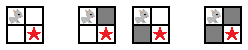The results of all $2$ x $2$ mazes are summarized below:

 black squares possible mazes with paths without paths 0 1 1 0 1 2 2 0 2 1 0 1 totals: 4 3 1

A $3$ x $3$ grid has $3^2 = 9$ total squares, and subtracting the start and finish squares gives a total of $3^2 - 2 = 7$ squares that can be either black or white.

There is ${7 \choose 0} = 1$ way to have a $3$ x $3$ maze with exactly $0$ black squares, which has a path.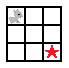There are ${7 \choose 1} = 7$ ways to have a $3$ x $3$ maze with exactly $1$ black square, and since $1$ square is not enough to block a path, all of those have paths.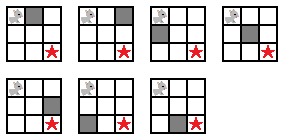There are ${7 \choose 2} = 21$ ways to have a $3$ x $3$ maze with exactly $2$ black squares, but there are only $2$ ways to block a path with just $2$ black squares - one to the right and one below the starting square, or one to the left and one above the finish square. This leaves $21 - 2 = 19$ possible mazes with paths.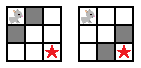There are ${7 \choose 3} = 35$ ways to have a $3$ x $3$ maze with exactly $3$ black squares, and analyzing this one is tougher. First of all, a path can be blocked with just $2$ squares in $2$ ways (as mentioned above), and there are $5$ other places to put the third square, for a total of $2 \cdot 5 = 10$ possible mazes without paths.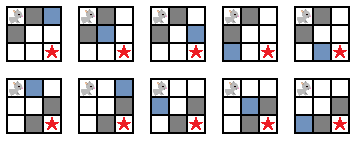Secondly, a path can be blocked with exactly $3$ squares (unique to the ones already mentioned) by making barriers from one side to an opposite corner in $4$ ways, from one side to an opposite side in $2$ ways, and from one corner to the other corner in $1$ way, for $7$ more ways.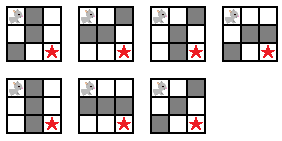Therefore, out of the total $35$ possible $3$ x $3$ mazes with exactly $3$ black squares, $10 + 7 = 17$ do not have paths, meaning $35 - 17 = 18$ do have paths.

There are ${7 \choose 4} = 35$ ways to have a $3$ x $3$ maze with exactly $4$ black squares. If the maze has $4$ black squares, then there must be $7 - 4 = 3$ white squares, and there are only $6$ ways of making a path with exactly $3$ white squares - $4$ that pass through the middle square, $1$ that passes through the top right corner square, and $1$ that passes through the bottom left corner square. Therefore, out of the total $35$ possible $3$ x $3$ mazes with exactly $4$ black squares, $6$ have paths, meaning $35 - 6 = 21$ do not.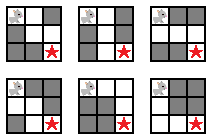There are ${7 \choose 5} = 21$ ways to have a $3$ x $3$ maze with exactly $5$ black squares. If the maze has $5$ black squares, then there must be $7 - 5 = 2$ white squares, which is not enough to complete a path from the starting square to the finishing square. Therefore all $21$ possible $3$ x $3$ mazes with exactly $5$ black squares do not have paths.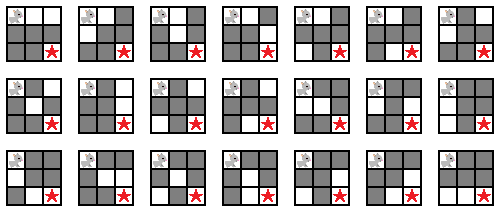By the same reasoning, all ${7 \choose 6} = 7$ ways of having a $3$ x $3$ maze with exactly $6$ black squares do not have possible paths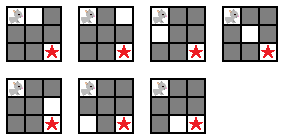and the ${7 \choose 7} = 1$ way to have a $3$ x $3$ maze with exactly $7$ black squares also does not have a possible path.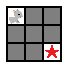Therefore, out of the total $2^7 = 128$ possible $3$ x $3$ mazes, $51$ have paths and $77$ do not. The results are summarized below:

 black squares possible mazes with paths without paths 0 1 1 0 1 7 7 0 2 21 19 2 3 35 18 17 4 35 6 29 5 21 0 21 6 7 0 7 7 1 0 1 totals: 128 51 77

As we move to larger mazes, the calculations needed become more and more complex. However, for now we can make a few generalizations:

For an $n$ x $n$ maze, there are $n^2 - 2$ squares that can be either black or white, and therefore $2^{n^2 - 2}$ total possible mazes.

All mazes need at least $2$ black squares to block a path. Therefore, the number of mazes with exactly $0$ and $1$ black squares always give paths. So the number $p$ of $n$ x $n$ mazes with paths is $\sum_{k = 0}^{1}{n^2 - 2 \choose k} < p < 2^{n^2 - 2}$.

All $n$ x $n$ mazes need at least $n - 1$ white squares in a path to go to the right side of the maze, and another $n - 2$ white squares in a path to go down to the bottom of the maze, for a total of at least $(n - 1) + (n - 2) = 2n - 3$ squares. If it has $2n - 4$ or less white squares, a path cannot be made. Therefore, the number $q$ of $n$ x $n$ mazes without paths is $\sum_{k = 0}^{2n - 4}{n^2 - 2 \choose k} < q < 2^{n^2 - 2}$.

- 3 years, 2 months ago

NOTE: I missed 18 squares. Check Stephan VDW's reply to this for the full list

A comprehensive list of non-paths for a $3$ x $3$ maze (I think). $\blacksquare$ represents the entrance/exit. O is for open and C is for closed. 2 stands for either open or closed. $\begin{bmatrix} \blacksquare & C & 2 \\ C& 2 &2 \\ 2& 2 & \blacksquare \end{bmatrix}$ giving $2^{5}$ possible versions. $\begin{bmatrix} \blacksquare & C & 2 \\ O & C &2 \\ C& 2 & \blacksquare \end{bmatrix}$ and its symmetrical equivalent giving $2 \cdot 2^{3}$. $\begin{bmatrix} \blacksquare & O & C \\ O & C &2 \\ C& 2 & \blacksquare \end{bmatrix}$ which gives $2^{2}$.

That is the end of the reusable ones. (1)

$\begin{bmatrix} \blacksquare & C & 2 \\ O & C &2 \\ O & C & \blacksquare \end{bmatrix}$ giving $2^{2}$. and its horizontal equivalent.

$\begin{bmatrix} \blacksquare & O & C \\ O & C &2 \\ O & C & \blacksquare \end{bmatrix}$ giving $2^{1}$. Note the symmetrical equivalent would close the 'door' to the maze and hence is not possible. and finally $\begin{bmatrix} \blacksquare & O & O \\ O & O &C \\ O & C & \blacksquare \end{bmatrix}$ giving just 1 ($2^{0}$ ).

Edit: The total is 59.

There is also an almost nice pattern if you 'overlay' the 2's. However I don't think this is useful in any way.

$\begin{bmatrix} \blacksquare & 0 & 2^{3}\\ 0& 2 & 2^5 \\ 2^{3} & 2^4 & \blacksquare \end{bmatrix}$

(1) Note we cannot reuse the following ones as the 'Go-around path' exists: $\begin{bmatrix} \blacksquare & C & & \\ | & C & & \\ | & C & & \\ \leftharpoonup & \rightarrow & \rightarrow & \blacksquare \end{bmatrix}$

- 3 years, 2 months ago

It seems like you missed a few. I like ordering by how many squares you can reach from the entrance (not counting the entrance itself) to get a complete list.

Exactly 0 squares: $\begin{bmatrix} \blacksquare & C & 2 \\ C & 2 & 2 \\ 2 & 2 & \blacksquare \end{bmatrix} = 32$

Exactly 1 square: $\begin{bmatrix} \blacksquare & O & C \\ C & C & 2 \\ 2 & 2 & \blacksquare \end{bmatrix} \text{ and symmetry } = 16$

Exactly 2 squares (you missed the last one): $\begin{bmatrix} \blacksquare & O & C \\ O & C & 2 \\ C & 2 & \blacksquare \end{bmatrix} = 4 \\ \begin{bmatrix} \blacksquare & C & 2 \\ O & C & 2 \\ O & C & \blacksquare \end{bmatrix} \text{ and symmetry } = 8 \\ \begin{bmatrix} \blacksquare & C & 2 \\ O & O & C \\ C & C & \blacksquare \end{bmatrix} \text{ and symmetry } = 4$

Exactly 3 squares (you missed the second and third): $\begin{bmatrix} \blacksquare & O & C \\ O & C & 2 \\ O & C & \blacksquare \end{bmatrix} \text{ and symmetry } = 4 \\ \begin{bmatrix} \blacksquare & C & 2 \\ O & O & C \\ O & C & \blacksquare \end{bmatrix} \text{ and symmetry } = 4 \\ \begin{bmatrix} \blacksquare & O & C \\ O & O & C \\ C & C & \blacksquare \end{bmatrix} = 1$

Exactly 4 squares (you missed both): $\begin{bmatrix} \blacksquare & O & O \\ O & O & C \\ C & C & \blacksquare \end{bmatrix} \text{ and symmetry } = 2 \\ \begin{bmatrix} \blacksquare & O & O \\ O & C & C \\ O & C & \blacksquare \end{bmatrix} = 1$

Exactly 5 squares: $\begin{bmatrix} \blacksquare & O & O \\ O & O & C \\ O & C & \blacksquare \end{bmatrix} = 1$

For a total of 77.

- 3 years, 2 months ago

A simpler version of the problem would be to only allow moving down and to the right. After thinking for some time about that simpler problem, I was able to figure out how to make a recursive algorithm that puts a range on the possible amount of solutions.

Let M(R,C) be a function that takes in the amount of rows (R) and the amount of columns (C) the grid has, and returns how many possible mazes there are. To get to the bottom-right, there has to be a path to the square one to the left of the exit, or one to the square above the exit.

It's quite easy to show M(1,C) = 1 for all C. The only way a path from the entrance to the exit can exist when there's only one row is when all the squares are open. Similarly, M(R,1) = 1 for all R.

When R and C are both at least 2, things get harder. There are three things that could happen:

1. The square to the left of the exit is open AND the square above the exit is closed. In this case the amount of paths to the exit is $M(R, C-1) * 2^{R-2}$. The $2^{R-2}$ is to account for the fact the rightmost column has R-2 squares that may either be open or closed freely without creating a new path, or destroying an old one.

2. The square to the left of the exit is closed AND the square above the exit is open, the amount of paths to the exit is $M(R-1, C) * 2^{C-2}$.

3. Both the square to the left of the exit and the square above the exit are open. This scenario is the hardest, because it's very easy to start double counting. This is a number that's at least $MAX( M(R,C-1) * 2^{C-2}, M(R-1,C) * 2^{R-2} )$ and at most $M(R,C-1) * 2^{C-2} + M(R-1,C) * 2^{R-2}$.

To elaborate how the bounds got determined, let's first look at the upper boundary. That one looks at all the paths to the exit and doesn't bother taking out the double counting. The lower boundary only looks at all the paths that come in from one direction and assumes the other direction is open even though it never gets used in a path.

Using the above math, the following table can get generated. But again, it's assumed it's only allowed to move down and to the right.

$\begin{array} {|l|l|l|l|l|l|} \hline R \ C & 1 & 2 & 3 & 4 & 5 \\ \hline 1 & 1 & 1 & 1 & 1 & 1 \\ \hline 2 & 1 & {[}3, 4{]} & {[}8, 12{]} & {[}20, 32{]} & {[}48, 80{]} \\ \hline 3 & 1 & {[}8, 12{]} & {[}48, 96{]} & {[}272, 640{]} & {[}1.472, 3.840{]} \\ \hline 4 & 1 & {[}20, 32{]} & {[}272, 640{]} & {[}3.264, 10.240{]} & {[}37.888, 143.360{]} \\ \hline 5 & 1 & {[}48, 80{]} & {[}1.472, 3.840{]} & {[}37.888, 143.360{]} & {[}909.312, 4.587.520{]} \\ \hline \end{array}$

- 3 years, 2 months ago

If only movements downwards and to the right are allowed, then $M(R,C)=\binom{R+C-2}{C-1}$

Despite this however, your recursive approach seems promising.

- 3 years, 2 months ago

What you describe is how many paths there are from the top-left to the bottom-right when all the squares are open. What I was trying to describe is how many unique mazes there are where at least one path exists.

To elaborate on the difference, let's look at the 2x2 grid. $\binom{R+C-2}{C-1} = \binom{2}{1} = 2$

To show there are more than 2 possible 2x2 mazes, here are three possible 2x2 mazes:

$\begin{bmatrix} O & O\\ O & O \end{bmatrix} \begin{bmatrix} O & X\\ O & O \end{bmatrix} \begin{bmatrix} O & O\\ X & O \end{bmatrix}$

An O represents an open square and an X represents a closed square. I hope this cleared up some confusion.

- 3 years, 2 months ago

- 3 years, 2 months ago

It might be easier to think of this in terms of the complement: How many arrangements do not have such a path? A grid will lack a path from corner to corner only if there is a barrier, a set of closed squares that completely encloses your starting point. A possible advantage of thinking in these terms is that any barrier on an order n grid is also a barrier on an order n+1 grid.

- 3 years, 2 months ago

An obvious approach to this problem would be to map out each unique path and configure the squares 'open and closed'. Trying to figure out which ones are the same is the only challenge then.

We also have the fact that each open square needs at least two open neighbors for a path to exist.

I'm guessing the paths are unique implies no double backing? i.e. a path A2 A3 A4 A3

- 3 years, 2 months ago

This could be a possible approach:

If we modify the rules to allow diagonal movements (movement along the diagonal), then if and only if the closed squares form a path from one side of the square to another, and passes by the top-left square, then there are no solutions to the maze.

Paths that the closed squares can form such that there are no solutions to the maze. These will be called barricades:Example of such a path:EDIT: As highlighted by Carl below, the significant advantage to this approach is that barricades for a smaller grid are also barricades for a larger grid. Perhaps a similar approach to Stefan (below), to consider a small grid of dimensions Rx1 and adding more collumns, can suffice.

- 3 years, 2 months ago

In your image if you add another row and column can you not just go around the barrier? Carl's point would only apply of you block both vertical and horizontal movement.

- 3 years, 2 months ago